# RD Sharma Solutions Class 11 Geometric Progressions Exercise 20.4

In this Exercise of RD Sharma Class 11 Chapter 20, we shall discuss problems based on the sum of an infinite G.P, with sample examples and illustrations for better understanding of the concepts. The solutions have been prepared by our expert faculty team to help students analyse their areas of weaknesses. RD Sharma Class 11 Maths Solutions is the best study material, which students can rely on and also build their confidence level in solving problems. It also provides strong conceptual knowledge among students to explore the key points in presenting a solution to a problem.

## Download the pdf of RD Sharma Solutions for Class 11 Maths Exercise 20.4 Chapter 20 – Geometric Progression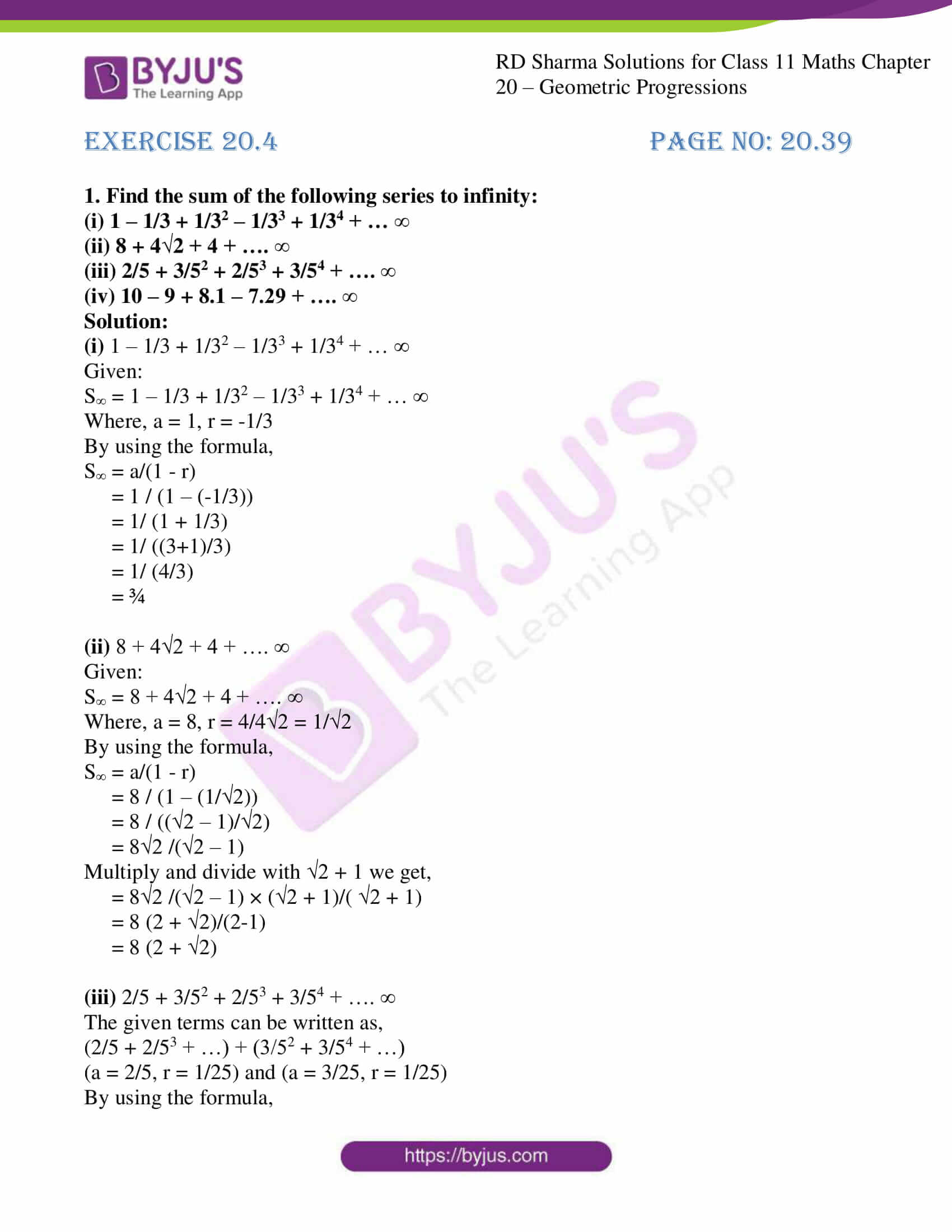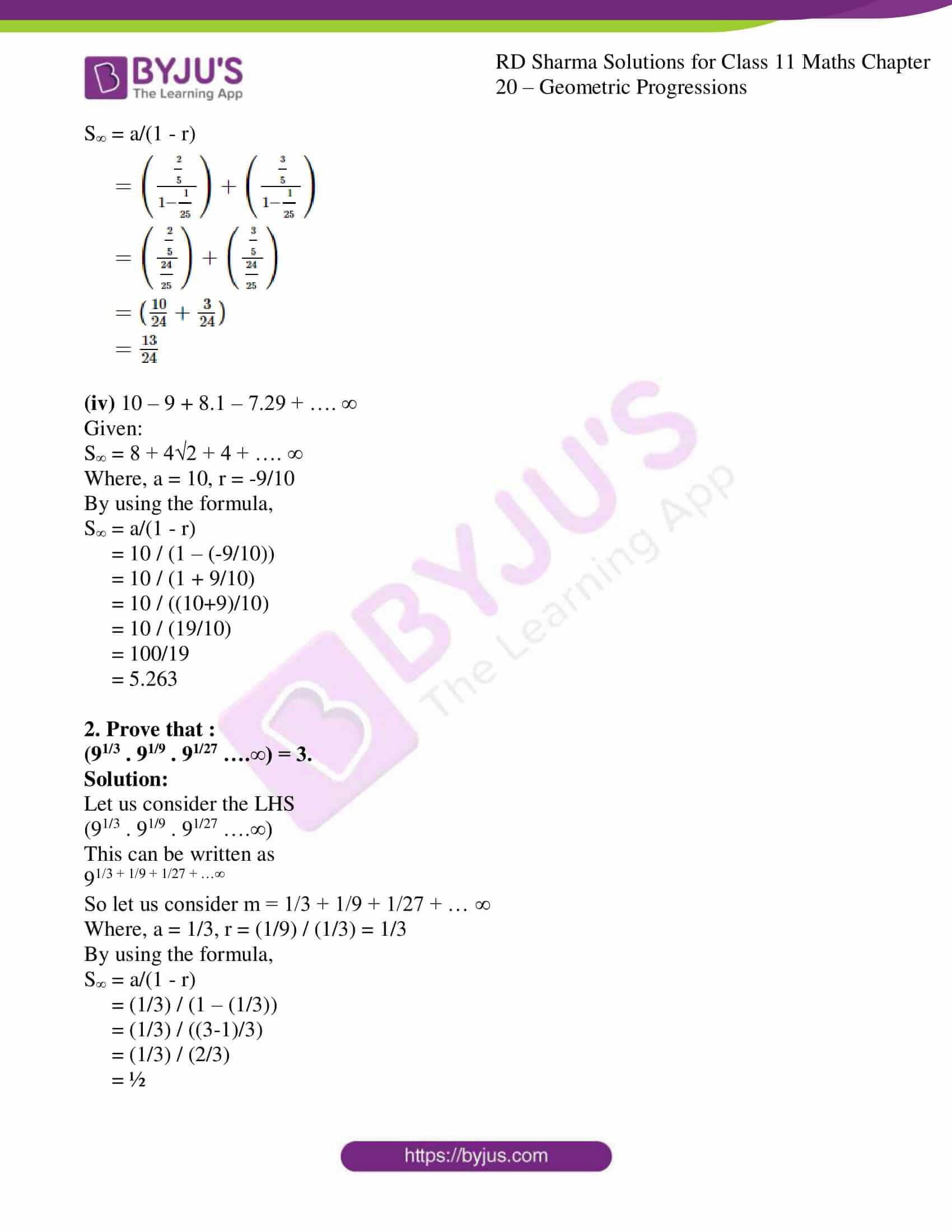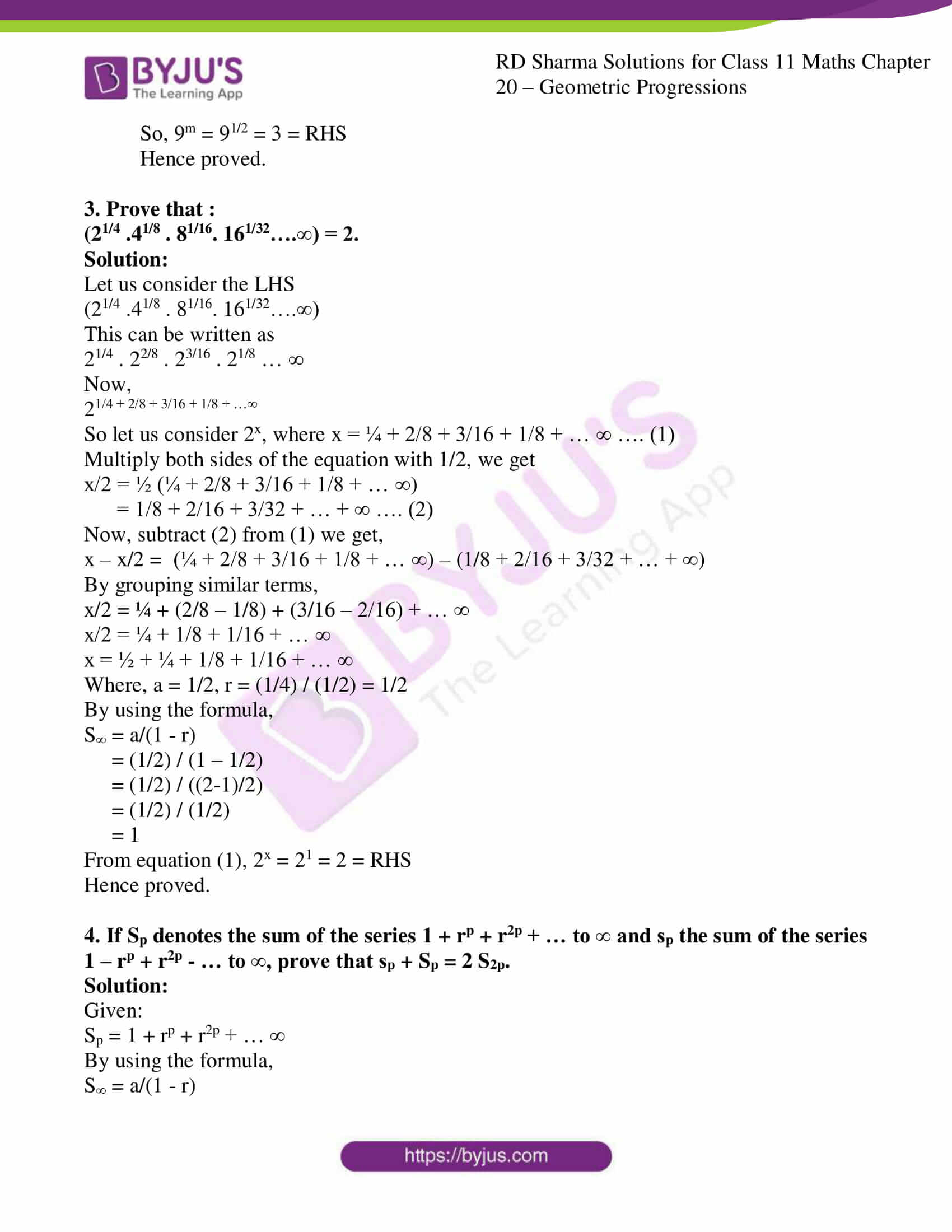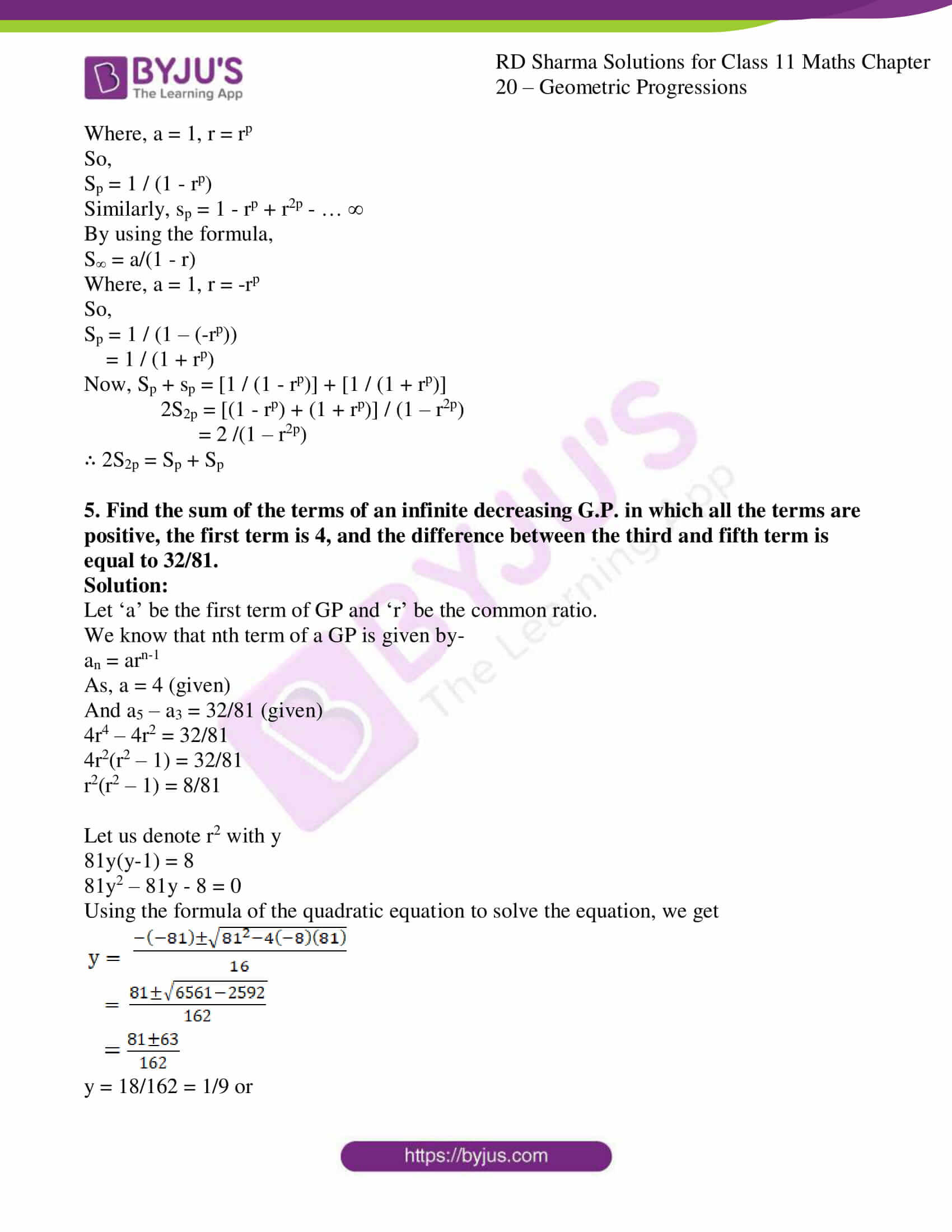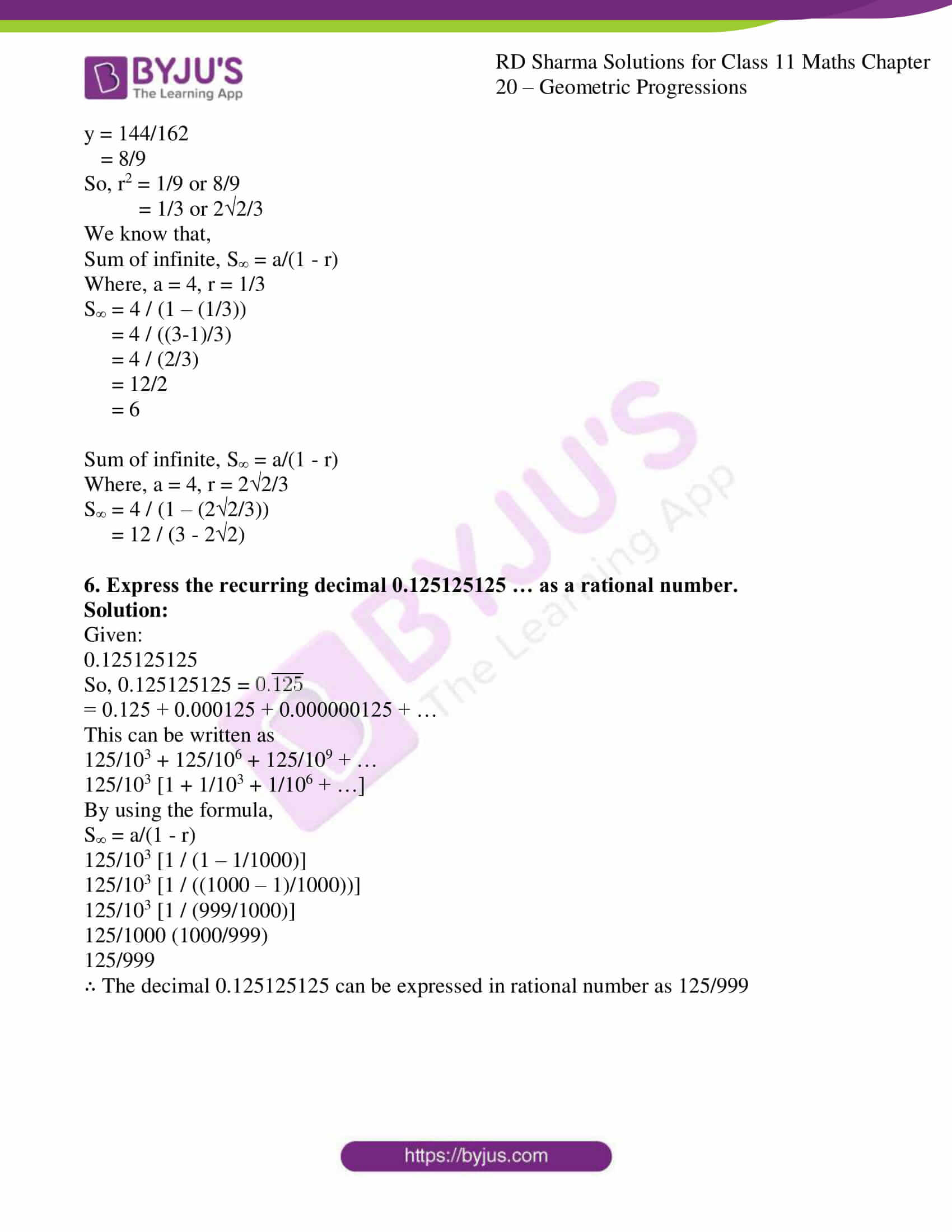### Access answers to RD Sharma Solutions for Class 11 Maths Exercise 20.4 Chapter 20 – Geometric Progression

1. Find the sum of the following series to infinity:

(i) 1 – 1/3 + 1/32 – 1/33 + 1/34 + … ∞

(ii) 8 + 4√2 + 4 + …. ∞

(iii) 2/5 + 3/52 + 2/53 + 3/54 + …. ∞

(iv) 10 – 9 + 8.1 – 7.29 + …. ∞

Solution:

(i) 1 – 1/3 + 1/32 – 1/33 + 1/34 + … ∞

Given:

S = 1 – 1/3 + 1/32 – 1/33 + 1/34 + … ∞

Where, a = 1, r = -1/3

By using the formula,

S = a/(1 – r)

= 1 / (1 – (-1/3))

= 1/ (1 + 1/3)

= 1/ ((3+1)/3)

= 1/ (4/3)

= ¾

(ii) 8 + 4√2 + 4 + …. ∞

Given:

S = 8 + 4√2 + 4 + …. ∞

Where, a = 8, r = 4/4√2 = 1/√2

By using the formula,

S = a/(1 – r)

= 8 / (1 – (1/√2))

= 8 / ((√2 – 1)/√2)

= 8√2 /(√2 – 1)

Multiply and divide with √2 + 1 we get,

= 8√2 /(√2 – 1) × (√2 + 1)/( √2 + 1)

= 8 (2 + √2)/(2-1)

= 8 (2 + √2)

(iii) 2/5 + 3/52 + 2/53 + 3/54 + …. ∞

The given terms can be written as,

(2/5 + 2/53 + …) + (3/52 + 3/54 + …)

(a = 2/5, r = 1/25) and (a = 3/25, r = 1/25)

By using the formula,

S = a/(1 – r)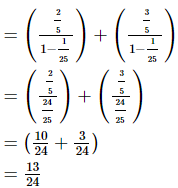(iv) 10 – 9 + 8.1 – 7.29 + …. ∞

Given:

S = 8 + 4√2 + 4 + …. ∞

Where, a = 10, r = -9/10

By using the formula,

S = a/(1 – r)

= 10 / (1 – (-9/10))

= 10 / (1 + 9/10)

= 10 / ((10+9)/10)

= 10 / (19/10)

= 100/19

= 5.263

2. Prove that :
(91/3 . 91/9 . 91/27 ….∞) = 3.

Solution:

Let us consider the LHS

(91/3 . 91/9 . 91/27 ….∞)

This can be written as

91/3 + 1/9 + 1/27 + …∞

So let us consider m = 1/3 + 1/9 + 1/27 + … ∞

Where, a = 1/3, r = (1/9) / (1/3) = 1/3

By using the formula,

S = a/(1 – r)

= (1/3) / (1 – (1/3))

= (1/3) / ((3-1)/3)

= (1/3) / (2/3)

= ½

So, 9m = 91/2 = 3 = RHS

Hence proved.

3. Prove that :

(21/4 .41/8 . 81/16. 161/32….∞) = 2.

Solution:

Let us consider the LHS

(21/4 .41/8 . 81/16. 161/32….∞)

This can be written as

21/4 . 22/8 . 23/16 . 21/8 … ∞

Now,

21/4 + 2/8 + 3/16 + 1/8 + …∞

So let us consider 2x, where x = ¼ + 2/8 + 3/16 + 1/8 + … ∞ …. (1)

Multiply both sides of the equation with 1/2, we get

x/2 = ½ (¼ + 2/8 + 3/16 + 1/8 + … ∞)

= 1/8 + 2/16 + 3/32 + … + ∞ …. (2)

Now, subtract (2) from (1) we get,

x – x/2 = (¼ + 2/8 + 3/16 + 1/8 + … ∞) – (1/8 + 2/16 + 3/32 + … + ∞)

By grouping similar terms,

x/2 = ¼ + (2/8 – 1/8) + (3/16 – 2/16) + … ∞

x/2 = ¼ + 1/8 + 1/16 + … ∞

x = ½ + ¼ + 1/8 + 1/16 + … ∞

Where, a = 1/2, r = (1/4) / (1/2) = 1/2

By using the formula,

S = a/(1 – r)

= (1/2) / (1 – 1/2)

= (1/2) / ((2-1)/2)

= (1/2) / (1/2)

= 1

From equation (1), 2x = 21 = 2 = RHS

Hence proved.

4. If Sp denotes the sum of the series 1 + rp + r2p + … to ∞ and sp the sum of the series 1 – rp + r2p – … to ∞, prove that sp + Sp = 2 S2p.

Solution:

Given:

Sp = 1 + rp + r2p + … ∞

By using the formula,

S = a/(1 – r)

Where, a = 1, r = rp

So,

Sp = 1 / (1 – rp)

Similarly, sp = 1 – rp + r2p – … ∞

By using the formula,

S = a/(1 – r)

Where, a = 1, r = -rp

So,

Sp = 1 / (1 – (-rp))

= 1 / (1 + rp)

Now, Sp + sp = [1 / (1 – rp)] + [1 / (1 + rp)]

2S2p = [(1 – rp) + (1 + rp)] / (1 – r2p)

= 2 /(1 – r2p)

∴ 2S2p = Sp + Sp

5. Find the sum of the terms of an infinite decreasing G.P. in which all the terms are positive, the first term is 4, and the difference between the third and fifth term is equal to 32/81.

Solution:

Let ‘a’ be the first term of GP and ‘r’ be the common ratio.

We know that nth term of a GP is given by-

an = arn-1

As, a = 4 (given)

And a5 – a3 = 32/81 (given)

4r4 – 4r2 = 32/81

4r2(r2 – 1) = 32/81

r2(r2 – 1) = 8/81

Let us denote r2 with y

81y(y-1) = 8

81y2 – 81y – 8 = 0

Using the formula of the quadratic equation to solve the equation, we get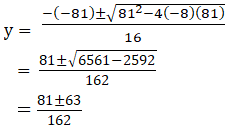y = 18/162 = 1/9 or

y = 144/162

= 8/9

So, r2 = 1/9 or 8/9

= 1/3 or 22/3

We know that,

Sum of infinite, S = a/(1 – r)

Where, a = 4, r = 1/3

S = 4 / (1 – (1/3))

= 4 / ((3-1)/3)

= 4 / (2/3)

= 12/2

= 6

Sum of infinite, S = a/(1 – r)

Where, a = 4, r = 22/3

S = 4 / (1 – (22/3))

= 12 / (3 – 22)

6. Express the recurring decimal 0.125125125 … as a rational number.

Solution:

Given:

0.125125125

So, 0.125125125 =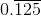= 0.125 + 0.000125 + 0.000000125 + …

This can be written as

125/103 + 125/106 + 125/109 + …

125/103 [1 + 1/103 + 1/106 + …]

By using the formula,

S = a/(1 – r)

125/103 [1 / (1 – 1/1000)]

125/103 [1 / ((1000 – 1)/1000))]

125/103 [1 / (999/1000)]

125/1000 (1000/999)

125/999

∴ The decimal 0.125125125 can be expressed in rational number as 125/999